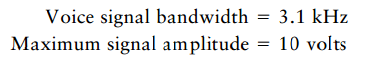Create an Account

Home / Questions / Consider a delta modulation DM system used to transmit a voice signal which is uniformly ...

Consider a delta modulation DM system used to transmit a voice signal which is uniformly sampled at the rate of 64 kHz Assume the following specifications a To avoid slope overload distortion

Consider a delta modulation (DM) system used to transmit a voice signal, which is uniformly sampled at the rate of 64 kHz. Assume the following specifications:(a) To avoid slope overload distortion, what is the minimum permissible value of the step size ∆ used in the system?

(b) Determine the average power of granular noise.

(c) Determine the minimum-channel bandwidth needed to transmit the DM encoded data.

Jul 31 2020 View more View LessSubscribe To Get Solution